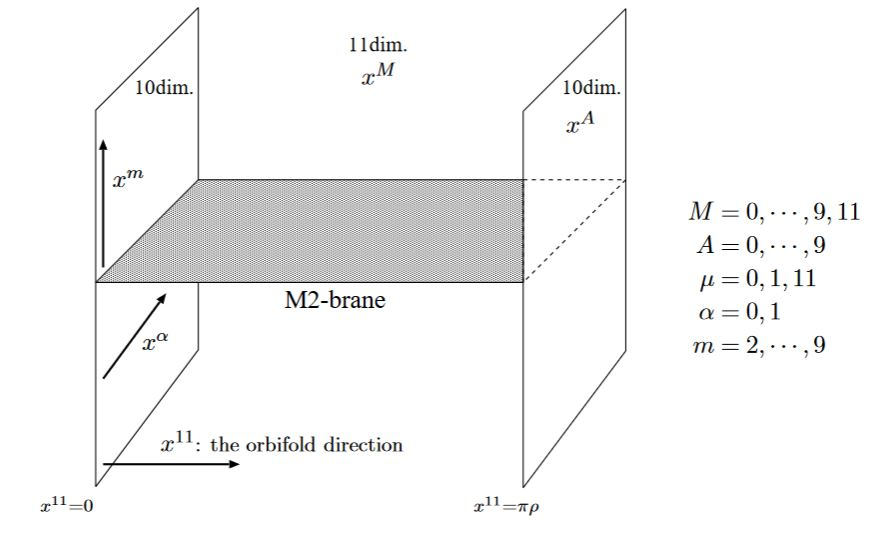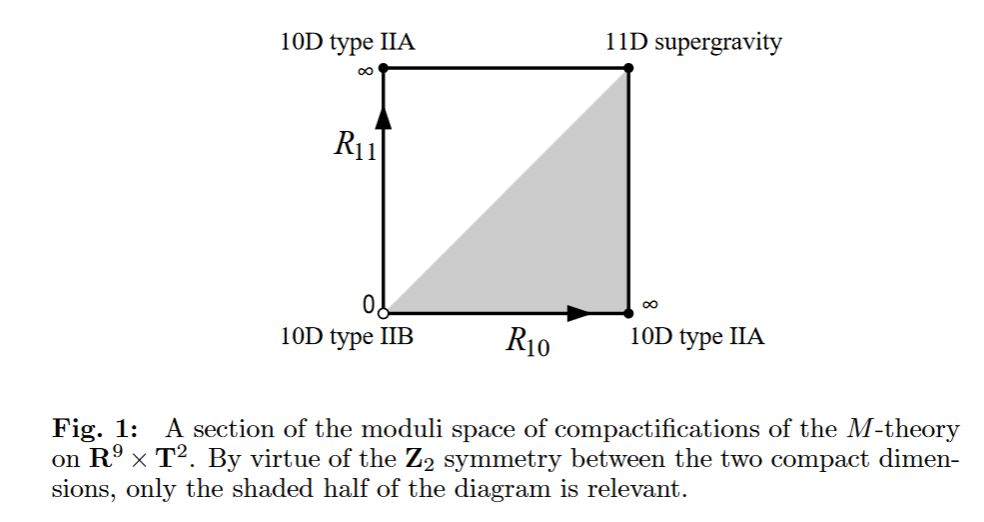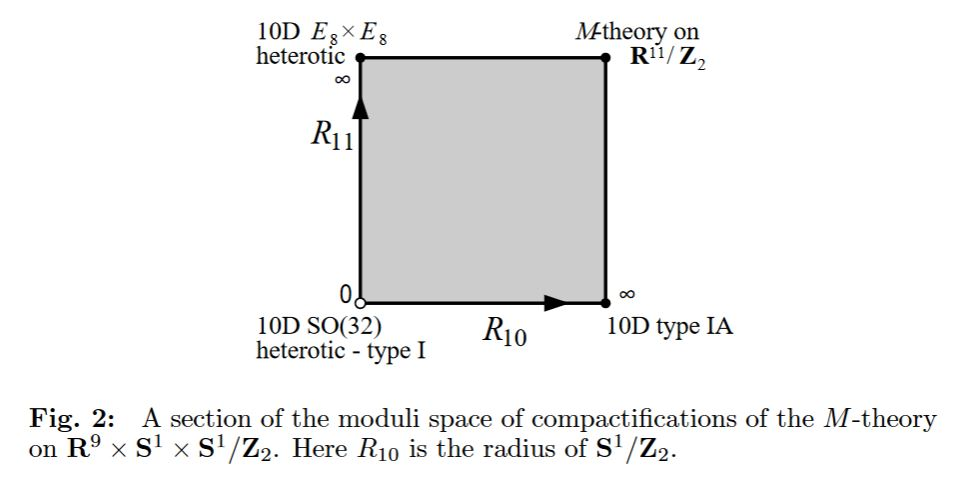# nLab Hořava-Witten theory

Contents

This entry is about the conjectured relation (duality) between M-theory at MO9-planes and heterotic string theory on these. For the relation of M-theory KK-compactified on a K3-surface and heterotic string theory on a 3-torus see instead at duality between M/F-theory and heterotic string theory.

### Context

#### Duality in string theory

duality in string theory

general mechanisms

string-fivebrane duality

string-string dualities

M-theory

F-theory

string-QFT duality

QFT-QFT duality:

# Contents

## Idea

There is an observation by Hořava–Witten 95, Hořava–Witten 96 which suggests that M-theory on an Z/2-orbifold (actually a higher orientifold) of the form $X_{10} \times (S^1 \sslash \mathbb{Z}_2))$ is “dual” to heterotic string theory on its boundary (fixed point) “M9-brane”. Therefore one also speaks of “heterotic M-theory” (Ovrut 02).from Kashima 00

In the above the circle factor is taken to be the circle fiber over the 10d type IIA spacetime.

If instead one considers another of the spatial dimensions to be compactified on $S^1\sslash \mathbb{Z}_2$, then, after T-duality (F-theory) result is supposed to be type I string theory. In this case the intersection of the M2-brane with the M9-brane (the latter now wrapping the M-theory circle fiber) is called the E-string.

More in detail:

One considers the KK-compactification of M-theory on a Z/2-orbifold of a torus, hence of the Cartesian product of two circles

$\array{ & S^1_A &\times& S^1_B \\ \text{radius}: & R_{11} && R_{10} }$

such that the reduction on the first factor $S^1_A$ corresponds to the duality between M-theory and type IIA string theory, hence so that subsequent T-duality along the second factor yields type IIB string theory (in its F-theory-incarnation). Now the diffeomorphism which exchanges the two circle factors and hence should be a symmetry of M-theory is interpreted as S-duality in type II string theory:

$IIB \overset{S}{\leftrightarrow} IIB$graphics taken from Horava-Witten 95, p. 15

If one considers this situation additionally with a $\mathbb{Z}/2\mathbb{Z}$-orbifold quotient of the first circle factor, one obtains the duality between M-theory and heterotic string theory (Horava-Witten theory). If instead one performs it on the second circle factor, one obtains type I string theory.

Here in both cases the involution action is by reflection of the circle at a line through its center. Hence if we identify $S^1 \simeq \mathbb{R} / \mathbb{Z}$ then the action is by multiplication by /1 on the real line.

In summary:

M-theory on

• $(S^1_A \sslash \mathbb{Z}_2 ) \times S^1_B$ yields heterotic string theory

• $S^1_A \times \left( S^1_B \sslash \mathbb{Z}_2 \right)$ yields type I' string theory

Hence the S-duality that swaps the two circle factors corresponds to a duality between heterotic E and type I’ string theory. And T-dualizing turns this into a duality between type I and heterotic string theory.

$\array{ HE &\overset{KK/\mathbb{Z}^A_2}{\leftrightarrow}& M &\overset{KK/\mathbb{Z}^B_2}{\leftrightarrow}& I' \\ \mathllap{T}\updownarrow && && \updownarrow \mathrlap{T} \\ HO && \underset{\phantom{A}S\phantom{A}}{\leftrightarrow} && I }$graphics taken from Horava-Witten 95, p. 16

### Duality between M-theory and heterotic string theory

Here each of the two copies of the heterotic gauge theory is a “hidden sector” with respect to the other.

The orbifold equivariance condition of the supergravity C-field is that discussed at orientifold (there for the B-field). Therefore it has to vanish at the two fixed fixed points of the $\mathbb{Z}_2$-action. Thereby the quantization condition

$[2G_4] = 2 [c_2] - [\frac{1}{2} p_1]$

on the supergravity C-field becomes the condition for the Green-Schwarz mechanism of the heterotic string theory on the “boundary” (the orbifold fixed points).

### Duality between M-theory and type I string theory

duality between M-theory and type I string theory

originally suggested in (Horava-Witten 95, section 3)

Further evidence is reviewed in APT 98

### Duality between heterotic and type I string theory

duality between heterotic and type I string theory

via S-duality in the F-theory-picture…

## Properties

### Boundary conditions

The supergravity C-field $\hat G_4$ is supposed to vanish, and differentially vanish at the boundary in the HW model, meaning that also the local connection 3-form $C_3$ vanishes there. The argument is roughly as follows (similar for as in Falkowski, section 3.1).

$C_3 \mapsto C_3 \wedge G_4 \wedge G_4$

in the Lagrangian of 11-dimensional supergravity is supposed to be well-defined on fields on the orbifold and hence is to be $\mathbb{Z}_2$-invariant.

Let $\iota_{11}$ be the canonical vector field along the circle factor. Then the component of $G \wedge G$ which is annihilated by the contraction $\iota_{11}$ is necessarily even, so the component $d x^{11}\wedge \iota_11 C_3$ is also even. It follows that also $d x^{11}\wedge \iota_11 G_4$ is even.

Moreover, the kinetic term

$C \mapsto G \wedge \star G$

is to be invariant. With the above this now implies that the components of $G$ annihiliated by $\iota_{11}$ is odd, because so is the mixed component of the metric tensor.

This finally implies that the restriction of $C_3$ to the orbifold fixed points has to be closed.

### General

The original articles are

Review is in

The black M2-brane solution in HW-theory, supposedly yielding the black heterotic string at the intersection with the M9-brane is discussed in

• Zygmunt Lalak, André Lukas, Burt Ovrut, Soliton Solutions of M-theory on an Orbifold, Phys. Lett. B425 (1998) 59-70 (arXiv:hep-th/9709214)

• Ken Kashima, The M2-brane Solution of Heterotic M-theory with the Gauss-Bonnet $R^2$ terms, Prog.Theor.Phys. 105 (2001) 301-321 (arXiv:hep-th/0010286)

More on the Green-Schwarz mechanism in Hořava-Witten theory:

Explicit discussion of worldvolume CFT of the M2-branes ending on the HW fixed points and becoming heterotic strings is discussed, via the BLG model, in

After KK-reduction to 5d supergravity there is a corresponding 5d mechanism, see the references there.

Disucussion of the duality between heterotic and type I string theory includes

• I. Antoniadis, H. Partouche, T.R. Taylor, Lectures on Heterotic-Type I Duality, Nucl.Phys.Proc.Suppl. 61A (1998) 58-71; Nucl.Phys.Proc.Suppl. 67 (1998) 3-1

Discussion of string phenomenology in Horava-Witten theory:

### Phenomenology

Discussion of phenomenology/string phenomenology for the heterotic M-theory, relating to the standard model of particle physics:

and with emphasis on heterotic line bundle-models:

### Gaugino condensation and supersymmetry breaking

Discussion of gaugino condensation and supersymmetry breaking in Horava-Witten theory, where the two heterotic MO9-branes provide a natural mechanism for supersymmetry breaking on one (the physical brane) by gaugino condensation on the other (the “dark sector” brane):

lecture notes:

original articles:

specifically for M-theory on S1/G_HW times H/G_ADE:

• Zygmunt Lalak, Steven Thomas, Gaugino Condensation, Moduli Potentials and Supersymmetry Breaking in M-Theory Models, Nuclear Physics B Volume 515, Issues 1–2, 30 March 1998, Pages 55-72 Nuclear Physics B (hep-th/9707223, doi:10.1016/S0550-3213(97)00784-0)

With emphasis on heterotic line bundles in the hidden sector:

• Anthony Ashmore, Sebastian Dumitru, Burt Ovrut, Section 4.2 of: Line Bundle Hidden Sectors for Strongly Coupled Heterotic Standard Models (arXiv:2003.05455)

• Anthony Ashmore, Sebastian Dumitru, Burt Ovrut, Explicit Soft Supersymmetry Breaking in the Heterotic M-Theory B−L MSSM (arXiv:2012.11029)

### Generalization to M-theory on $S^1/G_{HW} \times \mathbb{H}/G_{ADE}$

Generalization to M-theory on S1/G_HW times H/G_ADE:

### For 7d supergravity

Discussion for 7d supergravity:

• Tony Gherghetta, Alex Kehagias, Anomaly Cancellation in Seven-Dimensional Supergravity with a Boundary, Phys.Rev. D68 (2003), 065019, (arXiv:hep-th/0212060)

• Spyros D. Avramis, Alex Kehagias, Gauged $D=7$ Supergravity on the $S^1/\mathbb{Z}_2$ Orbifold (arXiv:hep-th/0407221)

• T.G. Pugh, Ergin Sezgin, Kellogg Stelle, $D=7$ / $D=6$ Heterotic Supergravity with Gauged R-Symmetry (arXiv:1008.0726)